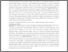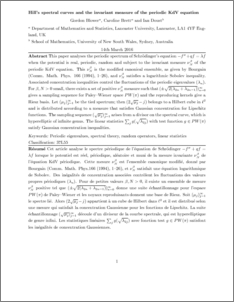# Hill's spectral curves and the invariant measure of the periodic KdV equation

Blower, Gordon and Brett, Caroline and Doust, Ian (2016) Hill's spectral curves and the invariant measure of the periodic KdV equation. Bulletin des Sciences Mathématiques, 140 (7). pp. 864-899. ISSN 0007-4497Preview
PDF (Hillconcentrationrevised)
Hillconcentrationrevised.pdf - Accepted Version

## Abstract

This paper analyses the periodic spectrum of Schrodinger's equation $-f''+qf=\lambda f$ when the potential is real, periodic, random and subject to the invariant measure $\nu_N^\beta$ of the periodic KdV equation. This $\nu_N^\beta$ is the modified canonical ensemble, as given by Bourgain ({Comm. Math. Ph899ys.} {166} (1994), 1--26), and $\nu_N^\beta$ satisfies a logarithmic Sobolev inequality. Associated concentration inequalities control the fluctuations of the periodic eigenvalues $(\lambda_n)$. For $\beta, N>0$ small, there exists a set of positive $\nu_N^\beta$ measure such that $(\pm \sqrt{2(\lambda_{2n}+\lambda_{2n-1})})_{n=0}^\infty$ gives a sampling sequence for Paley--Wiener space $PW(\pi )$ and the reproducing kernels give a Riesz basis. Let $(\mu_j)_{j=1}^\infty$ be the tied spectrum; then $(2\sqrt{\mu_j}-j)$ belongs to a Hilbert cube in $\ell^2$ and is distributed according to a measure that satisfies Gaussian concentration for Lipschitz functions. The sampling sequence $(\sqrt{\mu_j})_{j=1}^\infty$ arises from a divisor on the spectral curve, which is hyperelliptic of infinite genus. The linear statistics $\sum_j g(\sqrt{\lambda_{2j}})$ with test function $g\in PW(\pi)$ satisfy Gaussian concentration inequalities.\par

Item Type:
Journal Article
Journal or Publication Title:
Bulletin des Sciences Mathématiques
This is the author’s version of a work that was accepted for publication in Bulletin des Sciences Mathematiques. Changes resulting from the publishing process, such as peer review, editing, corrections, structural formatting, and other quality control mechanisms may not be reflected in this document. Changes may have been made to this work since it was submitted for publication. A definitive version was subsequently published in Bulletin des Sciences Mathematiques, 140, 7, 2016 DOI: 10.1016/j.bulsci.2016.03.005
Uncontrolled Keywords:
/dk/atira/pure/subjectarea/asjc/2600
Subjects:
Departments:
ID Code:
78871
Deposited By:
Deposited On:
14 Apr 2016 08:42
Refereed?:
Yes
Published?:
Published